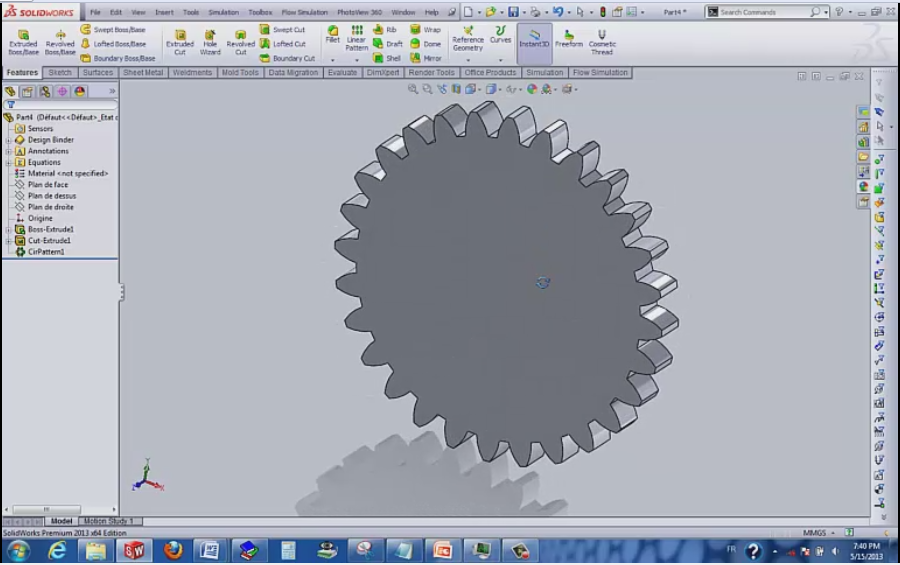# Tutorial: how to model '' a gear '' in '' solidworks '' and show design intent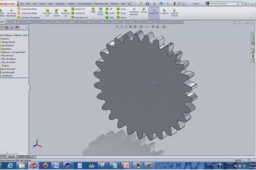1. ### Step 1:

01) File, new, part, ok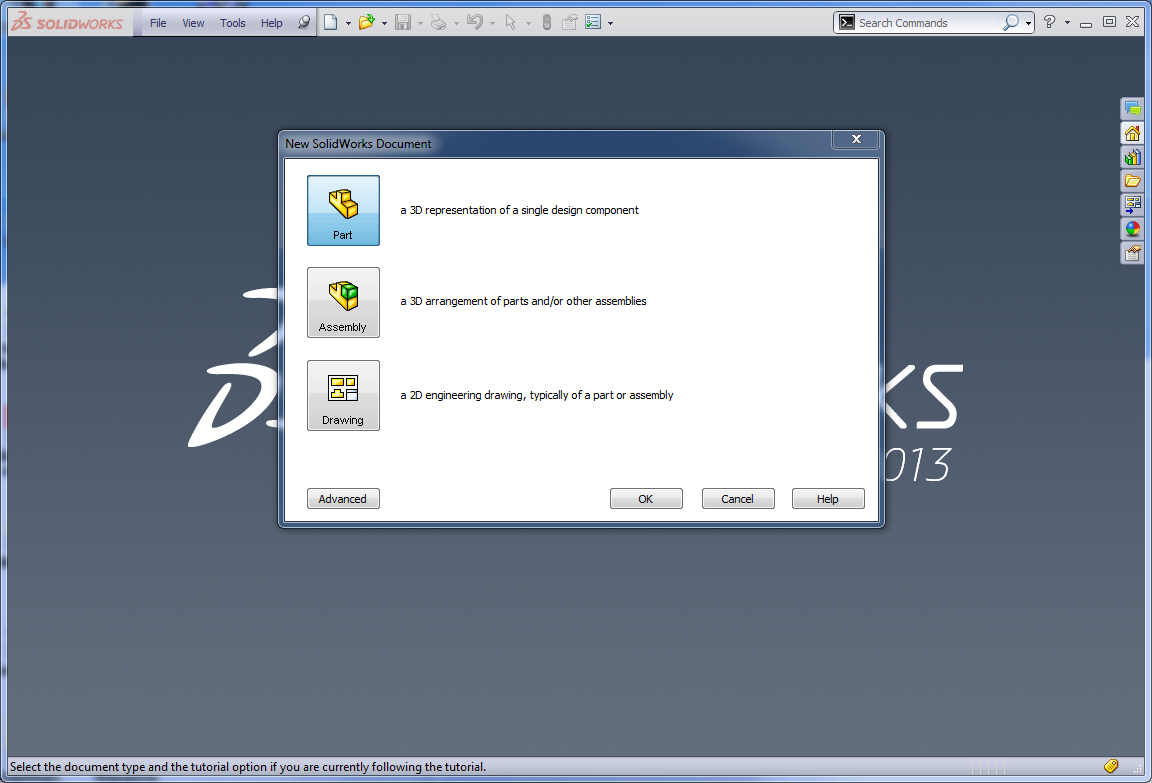2. ### Step 2:

02) Tools, equations, add these parameters and equations as shown
m= 6 (module)
z=20 (teeth number)
p= m*pi (pitch)
d=m*z (primitive diameter)
df=d-2*m (foot diameter)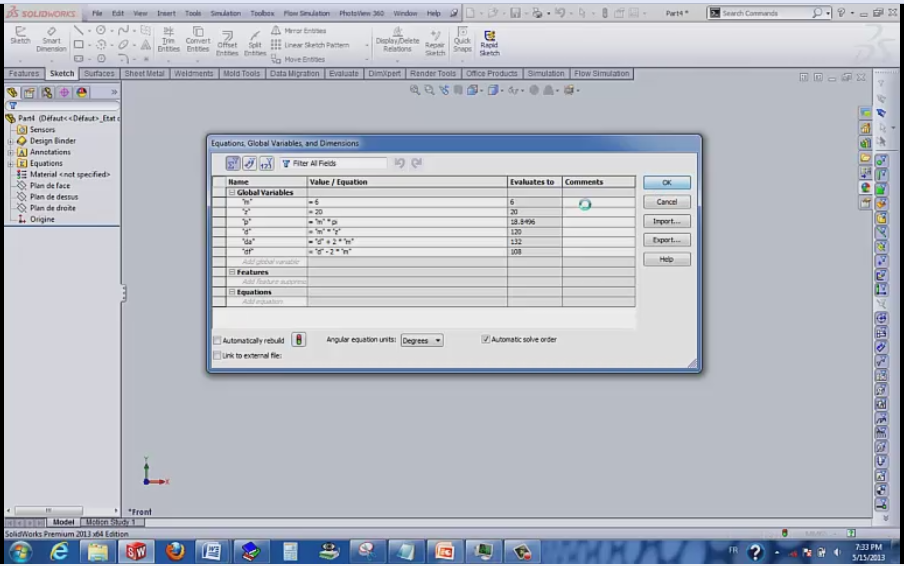3. ### Step 3:

03) Sketch, select front plan and draw a circle than
replace the dimension by “=” choose “da” (Rq, this is the same procedure for the rest.)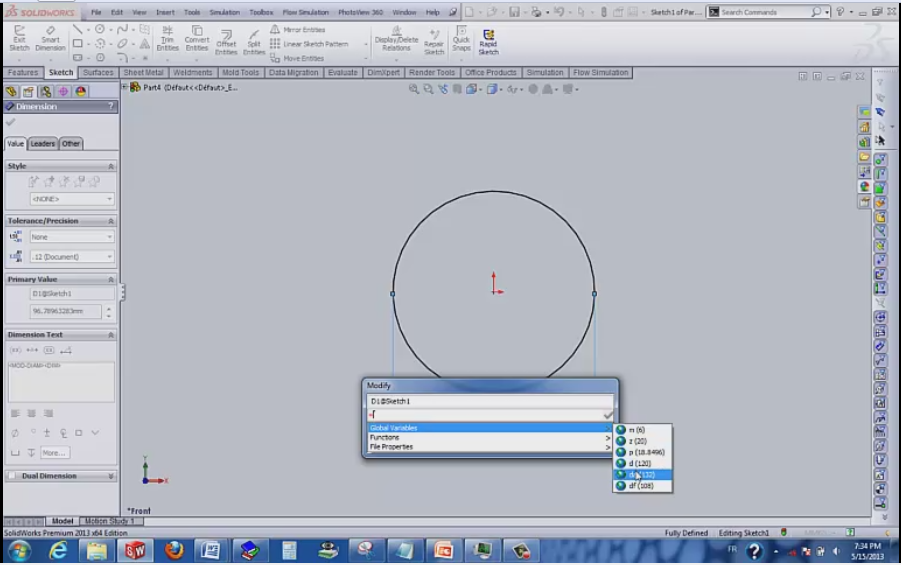4. ### Step 4:

04) Fuction, extrude, 25 for the thikness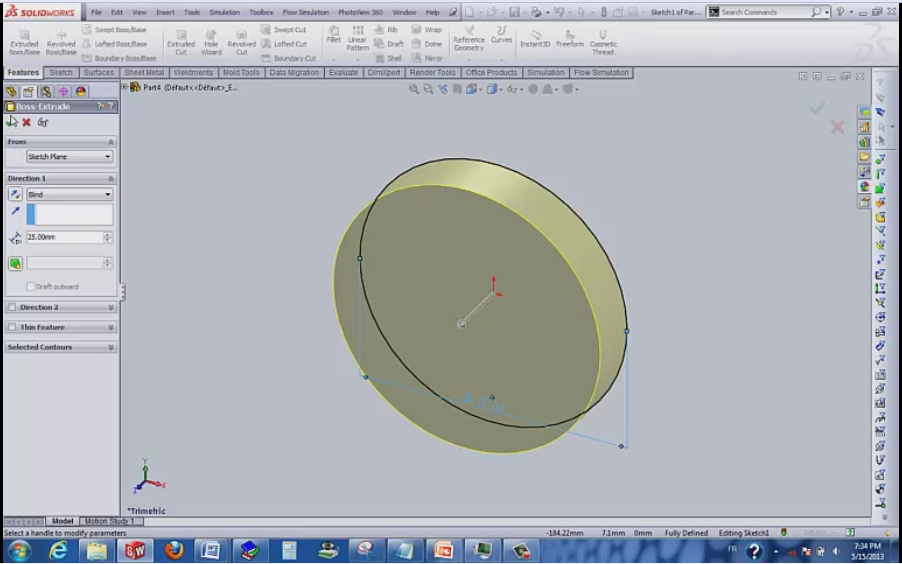5. ### Step 5:

05) A new sketch in the front face than add 3 circles and in the dimension box write “=” and chose :
The first circle: “df” the 2nd “d” the third “da”.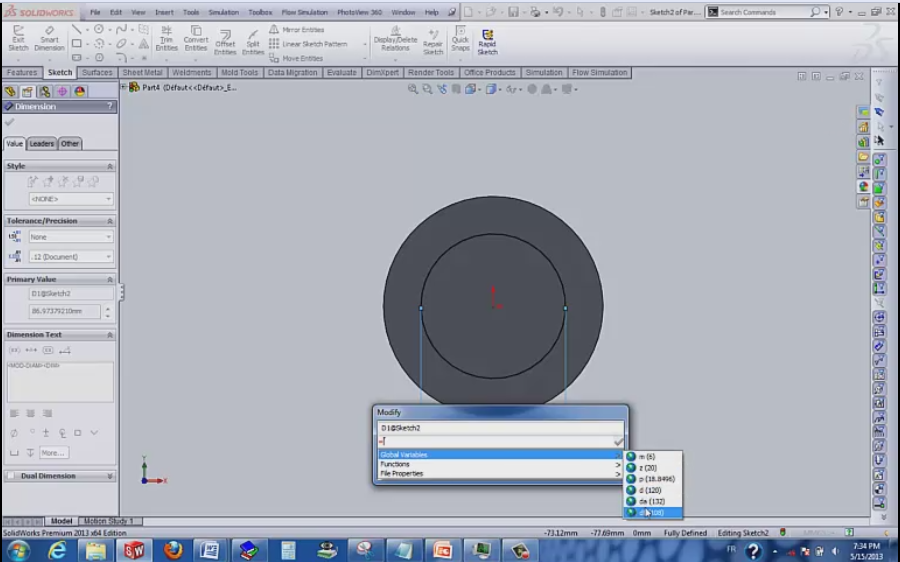6. ### Step 6:

06) Add 2 centerlines and mirror the 3rd one
Rq: Be sure that the end of the middle centerline coincide with the “d” circle.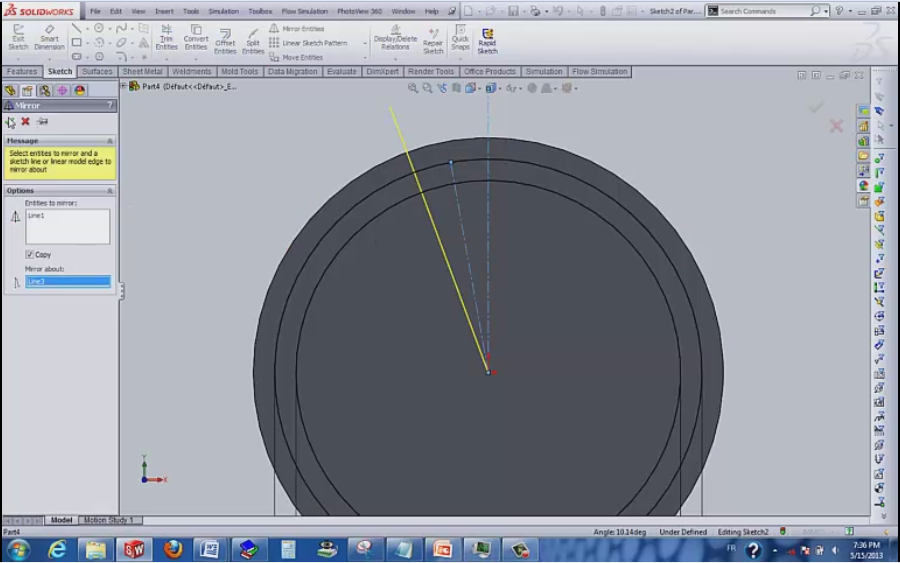7. ### Step 7:

07) Add an arc in as shown be sure always that the entities hang in the good positions.
The center in the “df” circle and and the ends on “da” and “df”
- Mirror it twice ( reference to the two centerlines)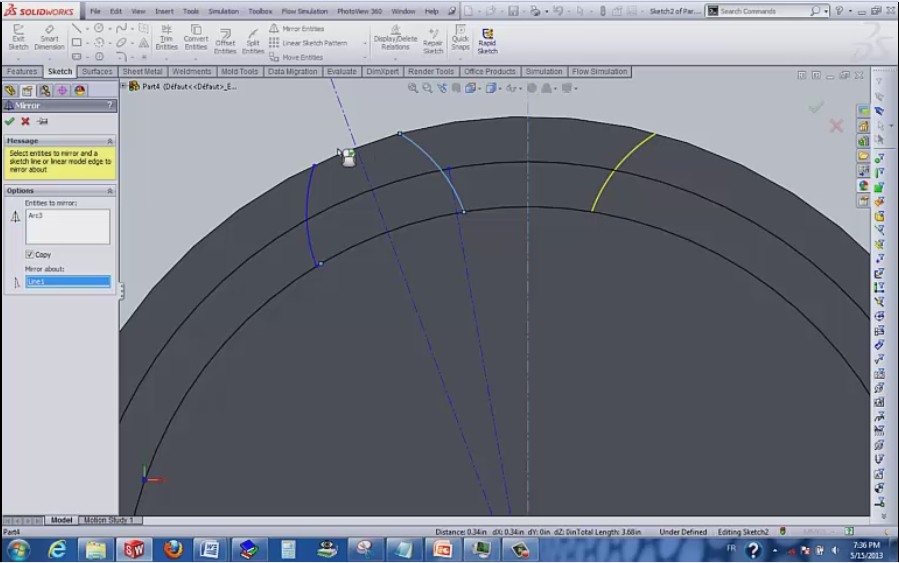8. ### Step 8:

08)
- and for the pitch use “=p”.
- and a relation between the arc and the point of the center line.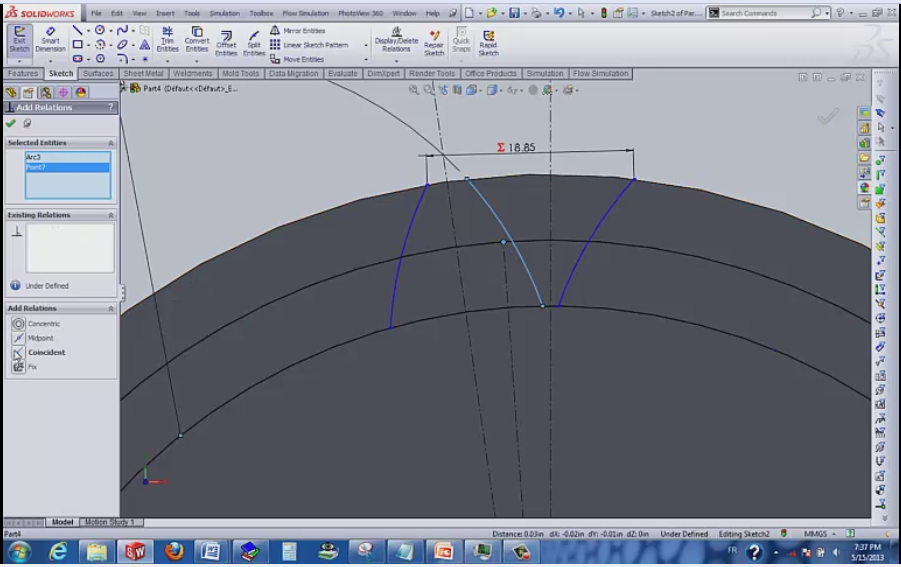9. ### Step 9:

09)
When the sketch is fully defined (black), extrude cut and select the two contours.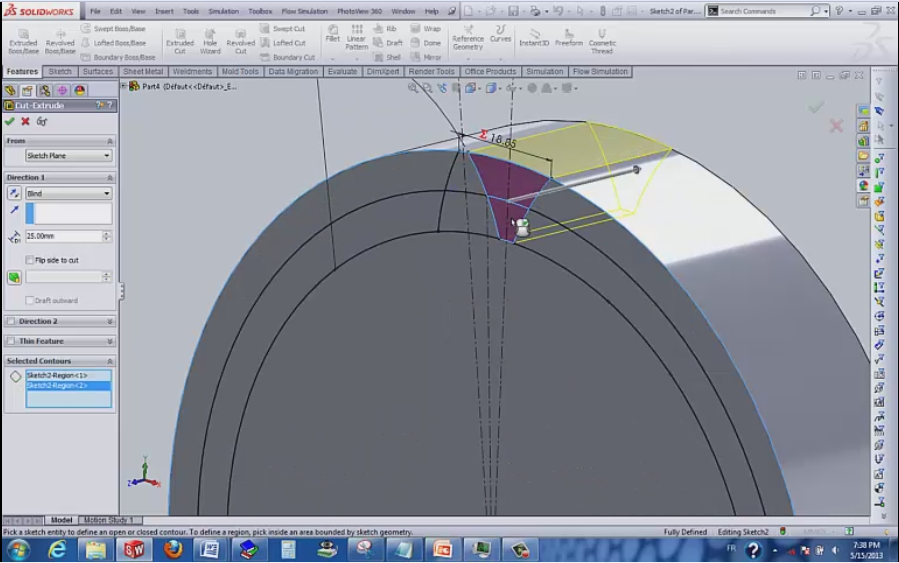10. ### Step 10:

10)
Use the circular pattern function and for the number of instance write “=” chose “z”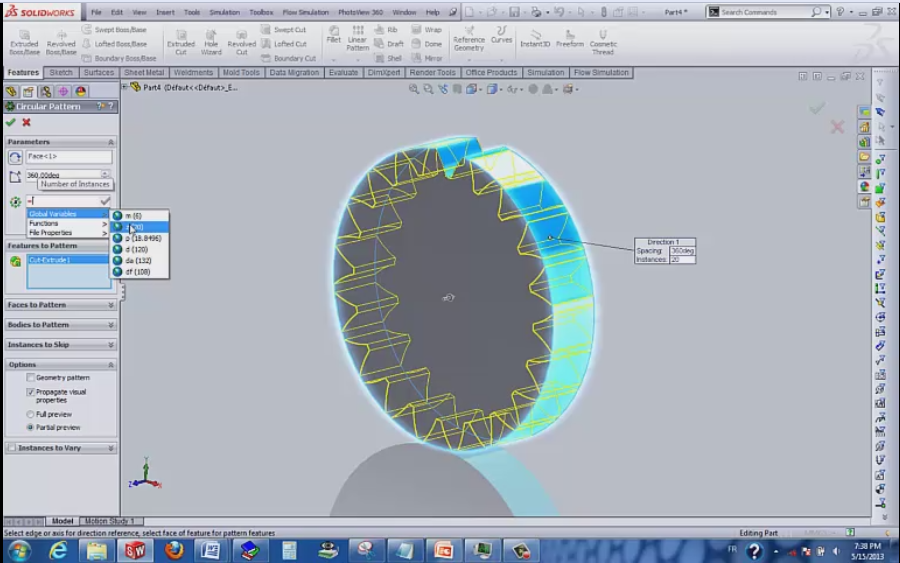11. ### Step 11:

And this is the result: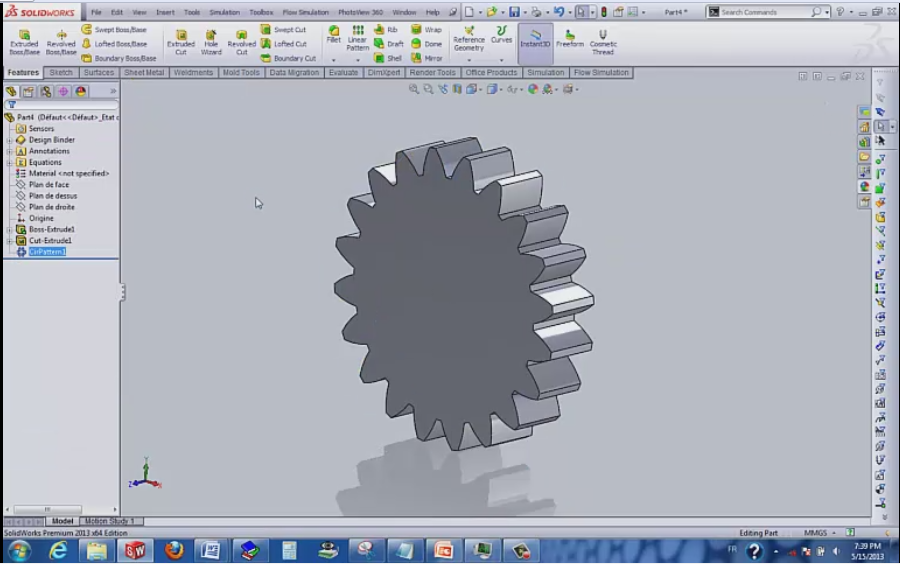12. ### Step 12:

11)
And now our goal is here to show the result of using the design intent ^^:
(tools, equations) We change the value of “m=9” and “z=28” for example.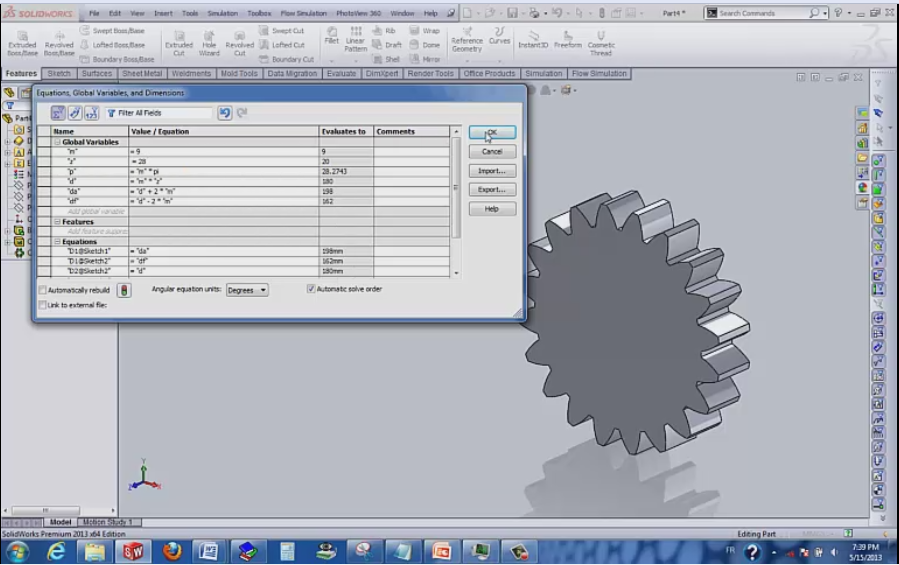13. ### Step 13:

12)
If you enjoy this tutorial be positive and click “it worked” and “like”